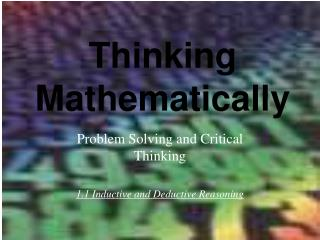DownloadDownload PresentationThinking Mathematically

# Thinking Mathematically

Download Presentation## Thinking Mathematically

- - - - - - - - - - - - - - - - - - - - - - - - - - - E N D - - - - - - - - - - - - - - - - - - - - - - - - - - -
##### Presentation Transcript

1. Problem Solving and Critical Thinking 1.1 Inductive and Deductive Reasoning Thinking Mathematically

2. Inductive Reasoning “Inductive reasoning” is the process of arriving at a general conclusion based on observations of specific examples. • Patterns • Conjecture / hypothesis • Counter-example

3. Inductive Reasoning There is no guarantee that the conclusions reached by “inductive reasoning” are correct with no exceptions. A strong inductive argument does not guarantee the truth of the conclusion, but rather provides strong support for the conclusion.

4. Inductive Reasoning In mathematics “inductive reasoning” is often used to find patterns. Exercise Set 1.1 #15, #23, #27 Identify a pattern, then use the pattern to find the next number: 1, 2, 4, 8, 16, __ 3, 6, 11, 18, 27, 38, __

5. Deductive Reasoning “Deductive reasoning” is the process of proving a specific conclusion from one or more general statements. A conclusion that is proved true by deductive reasoning is called a theorem. • Applying the rules of logic (chapter 3)

6. Inductive/Deductive Examples Inductive or Deductive? • We examine the fingerprints of 1000 people. No two individuals in this group of people have identical fingerprints. We conclude that for all people, no two people have identical fingerprints. • All mammals are warm-blooded animals. No snakes are warm-blooded. I have a pet snake. We conclude that my pet snake cannot be a mammal.

7. Counterexample If there is one case where for which a conjecture/hypothesis does not work, the conjecture is false. That one case is called a counterexample. • Example: Exercise Set 1.1 #3 If a number is multiplied by itself, the result is even. Disprove by counter-example.

8. Problem Solving and Critical Thinking 1.2 Estimation and Graphs Thinking Mathematically

9. “Rounding” Numbers To “round” to a particular place: Look at the digit to the right of the digit where rounding is to occur. 2. a. If the digit to the right is 5 or greater, add 1 to the digit to be rounded. Replace all digits to the right with zeros. b. If the digit to the right is less than 5, do not change the digit to be rounded. Replace all digits to the right with zeros.

10. Estimation in Calculations Exercise Set 1.2 #3, 11, 19, 21, 25 • Estimate e = 2.718281828459045 to the nearest thousandth. • Estimate 8.93 + 1.04 + 19.26 • Estimate 47.83 / 2.9 • Estimate 32% of 187,253 • A full-time employee who works 40 hours per week earns \$19.50 per hour. Estimate annual income.

11. Chapter 1: Problem Solving and Critical Thinking Thinking Mathematically